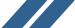PreCalculus Study Guide - Calculus Software, Calculus Homework, Calculus Help - Maplesoft
Included in Maple!

# Precalculus Interactive Study Guide

Prepare yourself for success in calculus.

##What is the Precalculus Study Guide?

The Precalculus Study Guide will help you master the math necessary for success in calculus. High school and university students in, or contemplating a calculus course, and students wanting additional insight into selected topics in algebra and trigonometry would all benefit from this guide.## Features

• Over 60 problems covering 11 topics from the introductory chapter of the typical calculus text
• 20 new Maplet tutors available only with the study guide
• Overviews of each topic
• Each problem is
• Solved the way it would be in a textbook
• Solved in a point-and-click fashion using specially designed Maplet tutors
• Solved with Maple commands, so you learn to unlock the complete power of Maple
• Hyperlinks to more than 140 definitions in the Maple Math Dictionary
• Over 175 additional exercises for further practice and deeper understanding
Textbook solution
Maplet tutor solution
Maple solution

##The Eleven Chapters Cover the Following Topic Areas:

1. Solving Inequalities
2. Graphing Lines in the Cartesian Plane
3. Graphing Rational Functions
4. The Arithmetic of Functions
5. Composition of Functions
6. Inverse Functions
7. The Elementary Transcendental Functions
8. Piecewise-Defined Functions
9. Functions Defined Implicitly